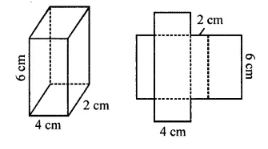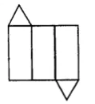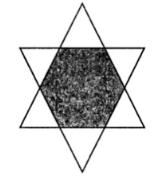# Selina Concise Solutions for Chapter 19 Representing 3-D in 2-D Class 8 ICSE Mathematics

##### Exercise 19

1.
If a polyhedron has 8 faces and 8 vertices, find the number of edges in it.
Solution
Faces = 8
Vertices = 8
using Eulers formula,
F + V – E = 2
⇒ 8 + 8 – E = 2
⇒ -E = 2 – 16
⇒ E= 14

2. If a polyhedron has 10 vertices and 7 faces, find the number of edges in it.
Solution
Vertices = 10
Faces = 7
Using Eulers formula,
F + V – E = 2
⇒ 7 + 10 – E = 2
⇒ -E = -15
⇒ E = 15

3. State, the number of faces, number of vertices and number of edges of:
(i) a pentagonal pyramid
(ii) a hexagonal prism
Solution
(i) A pentagonal pyramid
Number of faces = 6
Number of vertices = 6
Number of edges = 10

(ii) A hexagonal prism
Number of faces = 8
Number of vertices = 12
Number of edges = 18

4. Verily Euler’s formula for the following three dimensional figures:Solution
(i) Number of vertices = 6
Number of faces = 8
Number of edges = 12
Using Euler formula,
F + V – E = 2
⇒ 8 + 6 – 12 = 2
⇒ 2 = 2
Hence, proved.

(ii) Number of vertices = 9
Number of faces = 8
Number of edges = 15
Using, Euler’s formula,
F + V – E = 2
⇒ 9 + 8 – 15 = 2
⇒ 2 = 2
Hence, proved.

(iii) Number of vertices = 9
Number of faces = 5
Number of edges = 12
Using, Euler’s formula,
F + V – E = 2
⇒ 9 + 5 – 12 = 2
⇒ 2 = 2
Hence, proved.

5. Can a polyhedron have 8 faces, 26 edges and 16 vertices?
Solution
Number of faces = 8
Number of vertices = 16
Number of edges = 26
Using Euler’s formula
F + V – E
⇒ 8 + 16 – 26 ≠ -2
⇒ -2 ≠ 2
No, a polyhedron cannot have 8 faces, 26 edges and 16 vertices.

6. Can a polyhedron have:
(i) 3 triangles only?
(ii) 4 triangles only?
(iii) a square and four triangles?
Solution
(i) No
(ii) Yes
(iii) Yes

7. Using Euler’s formula, find the values of x, y, z.
 Faces Vertices Edges (i) x 15 20 (ii) 6 y 8 (iii) 14 26 z
Solution
(i) F + V – E = 2
⇒ x + 15 – 20 = 2
⇒ x – 5 = 2
⇒  = 2 + 5 = 7

(ii) F + V – E = 2
⇒ 15 + y – 26 = 2
⇒ y – 11 = 2
⇒ y = 2 + 11
⇒ y = 13

(iii) F + V – E = 2
⇒ 14 + 26 – Z = 2
⇒ -Z = 2 – 40
⇒ Z = 38

8. What is the least number of planes that can enclose a solid? What is the name of the solid.
Solution
The least number of planes that can enclose a solid is 4.
The name of the solid is Tetrahedron.

9. Is a square prism same as a cube?
Solution
Yes, a square prism is same as a cube.

10. A cubical box is 6 cm x 4 cm x 2 cm. Draw two different nets of it.
Solution11.
Dice are cubes where the sum of the numbers on the opposite faces is 7. Find the missing numbers a, b and c.Solution12. Name the polyhedron that can be made by folding each of the following nets:
(i)(ii)(iii)Solution
(i) Triangular prism. It has 3 rectangles and 2 triangles.
(ii) Triangular prism. It has 3 rectangles and 2 triangles.
(iii) Hexagonal pyramid as it has a hexagonal base and 6 triangles.

13. Draw nets for the following polyhedrons:Solution
Net of pentagonal pyramid:Net of pentagonal pyramid: